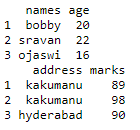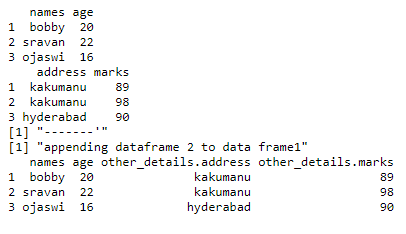# Append one dataframe to the end of another dataframe in R

• Last Updated : 07 Apr, 2021

In this article, we are going to append the data frame to another data frame using “\$” operator in R Programming Language.

Approach

• Create vectors
• Create one dataframe (dataframe1) by passing these vectors
• Create another dataframe (dataframe2)by passing these vectors
• Finally, append the dataframe2 to dataframe1 using” \$” operator.
• Display resultant dataframe

\$ operator is used to add dataframe as a column.

Syntax: dataframe_one\$column_name=dataframe_two

Parameters:

• column_name is the name of the new column
• dataframe_two is the dataframe 2 that is appended
• dataframe_one is the first dataframe.

Let us break the problem down into smaller clusters and make it is to understand, Let us first create two dataframes independently.

## R

 `# creating vectors for dataframe 1``names=``c``(``"bobby"``,``"sravan"``,``"ojaswi"``)``age=``c``(20,22,16)`` ` `# creating vectors for dataframe 2``address=``c``(``"kakumanu"``,``"kakumanu"``,``"hyderabad"``)``marks=``c``(89,98,90)`` ` `# pass these vectors to data frame1 a``a=``data.frame``(names,age)`` ` `# pass these vectors to data frame2 b``b=``data.frame``(address,marks)``print``(a)``print``(b)`

Output:After, two dataframes have been created let us append one into another.

## R

 `# creating vectors for dataframe 1``names=``c``(``"bobby"``,``"sravan"``,``"ojaswi"``)``age=``c``(20,22,16)`` ` `# creating vectors for dataframe 2``address=``c``(``"kakumanu"``,``"kakumanu"``,``"hyderabad"``)``marks=``c``(89,98,90)`` ` `# pass these vectors to data frame1 a``a=``data.frame``(names,age)`` ` `# pass these vectors to data frame2 b``b=``data.frame``(address,marks)`` ` `print``(a)``print``(b)``print``(``"-------'"``)``print``(``"appending dataframe 2 to data frame1"``)`` ` `# appending using \$ operator``a\$other_details=b``print``(a)`

Output:My Personal Notes arrow_drop_up